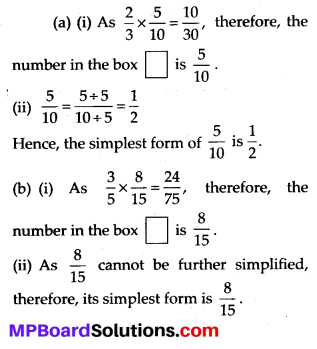# MP Board Class 7th Maths Solutions Chapter 2 Fractions and Decimals Ex 2.3

## MP Board Class 7th Maths Solutions Chapter 2 Fractions and Decimals Ex 2.3

Question 1.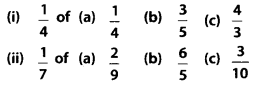Solution: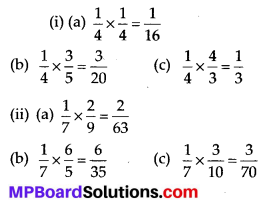Question 2.
Multiply and reduce to lowest form (if possible):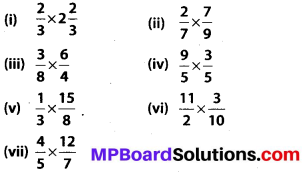Solution: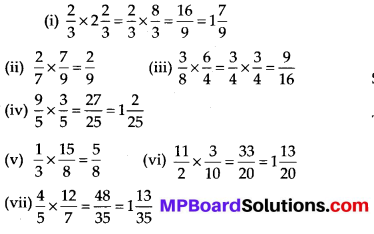Question 3.
Multiply the following fractions: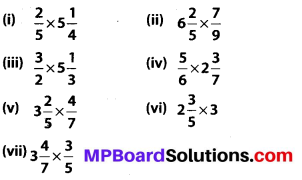Solution: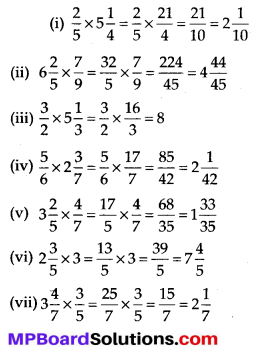Question 4.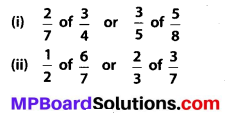Solution:
Converting these fractions into like fractions, we obtain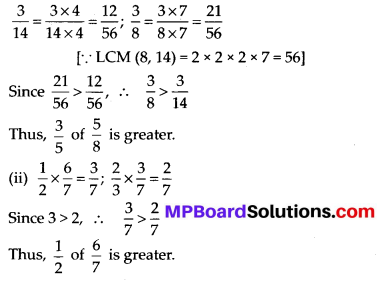Question 5.
Saili plants 4 saplings, in a row, in her garden. The distance between two adjacent saplings is $$\frac{3}{4}$$ m. Find the distance between the first and the last sapling.
Solution: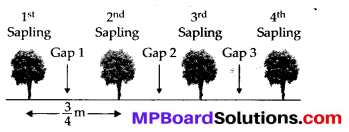From the figure, it can be observed that gap between first and last sapling = 3 × Length of gap 1
Therefore, distance between first and last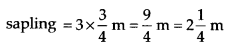Question 6.
Lipika reads a book for $$1 \frac{3}{4}$$ hours everyday. She reads the entire book in 6 days. How many hours in all were required by her to read the book?
Solution:
Time taken by Lipika to read the book per day = $$1 \frac{3}{4}$$ hours = $$\frac{7}{4}$$ hours
Number of days = 6
Total time taken by her to read the entire book = $$\frac{7}{4}$$ × 6 hours = $$\frac{21}{2}$$ hours
= $$=10 \frac{1}{2}$$hours

Question 7.
A car runs 16 km using 1 litre of petrol. How much distance will it cover using $$2 \frac{3}{4}$$ litres of petrol?
Solution:
A car can run per litre of petrol = 16 km
Quantity of petrol = $$2 \frac{3}{4}$$ litres = $$\frac{11}{4}$$ litres
So, a car can run for $$\frac{11}{4}$$ litres of petrol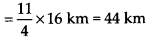Question 8.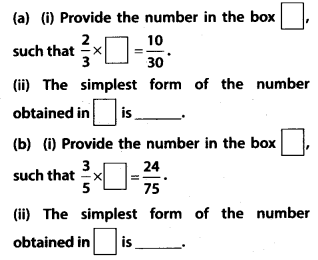Solution: